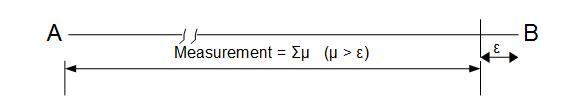# ijspace.org

 Incompleteness II 30th March 2014         Earlier we discussed the importance of zero-entropy measurement and how it relates to a stable or infinite life-time event. We can consider now a finite-segment AB of a line or curve in (t, x, y, z) space and ask ourselves how many points are there within this finite length? There is a requirement though, that we the observers should be able to measure a point accurately to the best of their abilities. That is where the limitation of the physical space asserts itself, as the smallest length measured (let us call it µ) no matter how small, would still be finite.      So what happens when we measure the segment AB? The situation is as shown below:No matter how accurate we are, there is always a finite ε ( > 0) which is less than µ and hence AB can not be fully measured. If we extend AB to complete the circle then there will be always some ε > 0 which can not be determined. So how would we know if the measured structure is a true circle? It could easily have been a spiral, or a slinky if you may, or some other shape. Therefore an observer of finite capability, we shall call it ObsM, either can not determine AB completely or it has to make an infinite number of measurements to determine AB absolutely. If a measurement made in determining AB is a PE1 measurement then i. it can not be a negative number, ii. it can not be an irrational number, iii. the definition of the origin is included, iv. AB can be measured completely or (µ < ε, ε = 0). We already know that a macroscopic observer ObsM can not make a PE1 measurement and hence the measurement set i. contains negative numbers, ii. contains irrationals, iii. does not contain the definition of origin, iv. (µ  > ε, ε > 0) and therefore AB can not be measured completely. In other words the measurement set for ObsM is represented by the real set R without the definition of null. This makes the zero of the set R a "weak" zero. It will follow VTS and represent the discrete measurement space or j-space. Therefore we can say that for a given PE1 event (we can think universe) the measurements made by observers come in only two flavors: 1. the single element set or the unit set {1} where the definition of origin is established, the case of an observer with infinite capability, 2. the infinite set R with a "weak" zero where the definition of the origin is not established, the case of an observer with finite capability or ObsM.       The element 0 in set R or Z is always a "weak" zero in j-space which exists to begin with only because null can not be truly defined. If null could have been defined than we will have a single element set with the element representing a PE1 measurement. Here we can also make a conjecture that this single number being a PE1 event can be a prime number. More on it later.       We may want to reflect upon Cantor's continuum hypothesis also known as CH, which states that there exist no infinite set whose cardinality is between set of integers Z and set of real numbers R. The cardinality represents the number of elements in a given set. We note that both Z and R are infinite sets in their own rights. For a PE1 measurement either Z or R if they exist, they exist because null can not be determined accurately due to observer's limitations or equivalently ε > 0. Therefore if null can not be determined the sets even if they are infinite represent incomplete information about a PE1 state. We seem to have run into a paradox where we are trying to determine a result (comparison of cardinalities) based on systems (Z or R) which represent incomplete information in measurement space. Essentially we are trying to define different levels of infinities which in j-space may be thought of as observers with different capabilities but with inherent entropies which will not allow the measurements to be completed (and hence infinities).       To sum up if we could measure complete information or equivalently there was no entropy (or µ < ε, ε = 0) then neither Z set nor R set would exist. If they do exist then it means that entropy is in picture (µ > ε,  ε = 0), and all the information is not available. Therefore we can not conclusively say that if Z and R exist than other infinite sets will not exist. We can not make such a definitive statement unless we have complete information which represents ε = 0 condition.       However what we can say is that all these sets will have to represent the same PE1 event represented by the unit set {1} because all these infinite sets exist within the universe and the universe is a PE1 event by assumption. Hence if the entropy could be eliminated and measurements could be completed than all the infinite sets (Z, R, and others) in j-space will converge to the unit set {1}.        We would also like to recommend the Statistical Mechanics by Shang-Keng Ma. The concept of entropy and how to think of the concept of force associated with it, are described in first few pages in most simple terms, a truly remarkable book.Information on www.ijspace.org is licensed under a Creative Commons Attribution 4.0 International License.